Short articles on topics in systematic trading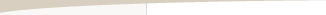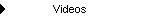Other Articles...Trade Dependency

by Michael R. Bryant

A runs test can be used to determine if there is any statistically significant dependency in the current sequence of trades. A runs test determines if the runs of wins and losses are significantly longer or shorter than can be expected if the trades occurred in random order. A "run" is simply a consecutive series of wins or losses. For example, suppose the trade sequence consists of \$500, \$200, \$300, \$150, -\$750, -\$250, \$350, \$400, -\$500. This series of nine trades consists of two runs of wins and two runs of losses. The first run of wins is four trades in length, which is followed by a run of two losses. Following that is a run of two wins and finally a run of one loss.

The runs test is a specialized z test that takes the following form:where r is the number of runs, W is the number of winning trades, L is the number of losing trades, X = (2WL)/(W + L), and N is the total number of trades (N = W + L).

The critical value of the z statistic comes from the z, or normal, distribution. With 95% confidence, for example, the critical value for a two-tailed test is 1.96. So, if the calculated z statistic is greater than 1.96 or less than -1.96, we would conclude that the number of runs is significant with 95% confidence. Positive values of z imply more runs than by chance; negative z values imply fewer runs than by chance.

If there are fewer runs than expected by chance, this implies the runs are longer than expected, which suggests positive dependency (wins follow wins; losses follow losses). If there are more runs than by chance, this implies the runs are shorter than expected, which suggests negative dependency (wins follow losses and losses follow wins). If the dependency has a high degree of significance (high confidence level) -- e.g., 95% or greater -- it may be worth exploiting.

There are several ways to exploit significant levels of trade dependency:Skip trades after a loss until a skipped trade would have been a winner. This rule can improve trading performance in cases of positive dependency where wins tend to follow wins and losses tend to follow losses.Skip the next trade after a win; take the next trade after a loss. This is intended to be used when wins tend to follow losses and losses tend to follow wins; i.e., negative dependency.After X wins in a row, skip the next Y trades. For example, if the average winning run is 3 trades, and average losing run is 2 trades, you might try skipping the next two trades after three wins in a row.After X losses in a row, skip the next Y trades. If, for example, there is positive dependency (so that losses tend to follow losses) and the average length of the losing runs is 4 trades, then you might try skipping the next three trades after the first loss.

Dependency rules such as these should only be applied when the dependency is statistically significant; for example, when the confidence level is 95% or greater. Over a long series of trades, significant levels of dependency are rare. However, over shorter trade sequences, it's not unusual to find significant dependency.

If you recalculate the dependency after each trade using a sliding window of trades -- say, over the last 30 trades -- you'll see the dependency change from trade to trade. It would not be unusual to see the dependency change from positive to negative and back several times over a long series of trades, with several periods of significant dependency. Under these circumstances, it would be worth trying to exploit the dependency when it's significant.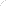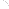Join Our Email List Email: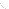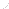For Email Marketing you can trust